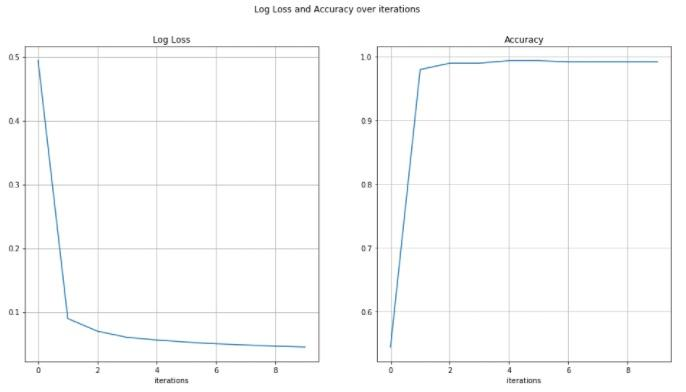USE Jupyter Notebook

Given Code: ‘Part of answer’ but incomplete…..

``````# Importing libraries
import pandas as pd
import numpy as np
from sklearn.neighbors import KNeighborsClassifier
from sklearn.tree import DecisionTreeClassifier
from sklearn.model_selection import train_test_split
from sklearn import metrics

# Separating features and target
features = data[['Pregnancies', 'Glucose', 'BloodPressure', 'SkinThickness', 'Insulin', 'BMI', 'DiabetesPedigreeFunction', 'Age']]
target = data['Outcome']

# Splitting training and testing data in the ratio 70 : 30 respectively
x_train, x_test, y_train, y_test = train_test_split(features, target, random_state=0, test_size=0.3)

# KNN model
modelKNN = KNeighborsClassifier()

# Train the model
modelKNN.fit(x_train, y_train)

# Predict test set
y_pred_knn = modelKNN.predict(x_test)

# Print accuracy of the KNN model
print(modelKNN.score(x_test,y_test))

# Print Confusion matrix
print(metrics.confusion_matrix(y_test, y_pred_knn))

# Print Classification Report for determining precision, recall, f1-score and support of the model
print(metrics.classification_report(y_test, y_pred_knn))

# Decision Tree model
modelDT = DecisionTreeClassifier()

# Train the model
modelDT.fit(x_train, y_train)

# Predict test set
y_pred_dt = modelDT.predict(x_test)

# Print accuracy of the Decision Tree model
print(modelDT.score(x_test,y_test))

# Print Confusion matrix
print(metrics.confusion_matrix(y_test, y_pred_dt))

# Print Classification Report for determining precision, recall, f1-score and support of the model
print(metrics.classification_report(y_test, y_pred_dt))``````

Please do the data cleaning and feature selection…

Please use the given code/layout given below original question….

ORIGINAL QUESTION:

Fill out the code below so that it creates a multi-layer perceptron with a single hidden layer (with 4 nodes) and trains it via back-propagation. Specifically your code should:

1. Initialize the weights to random values between -1 and 1
2. Perform the feed-forward computation
3. Compute the loss function
4. Calculate the gradients for all the weights via back-propagation
5. Update the weight matrices (using a learning_rate parameter)
6. Execute steps 2-5 for a fixed number of iterations
7. Plot the accuracies and log loss and observe how they change over time

First, initialise the parameters. This means determining the following:

Size of the network

Hidden layers (or a single hidden layer in this example) can be any size. Layers with more neurons are more powerful, but also more likely to overfit, and take longer to train. The output layer size corresponds to the number of classes.

Number of iterations

This parameter determines how many times the network will be updated.

Learning rate

Each time we update the weights, we do so by taking a step into the direction that we calculated will improve the accuracy of the network. The size of that step is determined by the learning rate. Taking small steps will slow the process down, but taking steps that are too large can cause results to vary wildly and not reach a stable optimum.

Next, fill in the code below to train a multi-layer perceptron and see if it correctly classies the input.

in[]# Reshape y
y =

# Initializing weights

W_1 =
W_2 =

# Definining number of iterations and learning rate(‘lr’)
num_iter =

learning rate =

in[]# Creating empty lists for loss values(error) and accuracy
loss_vals, accuracies = [], []

for j in range(num_iter):
# Do a forward pass through the dataset and compute the loss

# Decide on intervals and add on the current loss and accuracy to the respective list

# Update the weights

in[]# Plot the loss values and accuracyHigher number of iterations and lower learning rate seems to improve accuracy

## "Looking for a Similar Assignment? Get Expert Help at an Amazing Discount!"